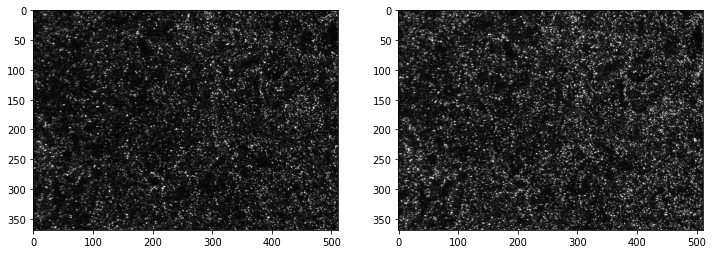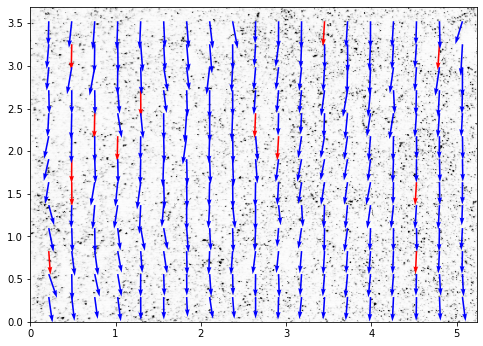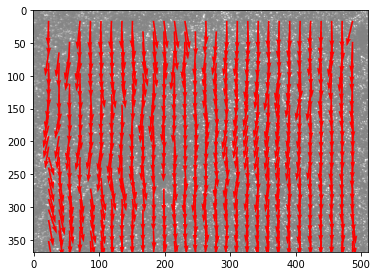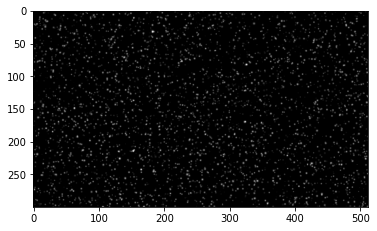# OpenPIV tutorial 1¶

In this tutorial we read the pair of images using imread, compare them visually and process using OpenPIV. Here the import is using directly the basic functions and methods

In :
from openpiv import tools, pyprocess, validation, filters, scaling

import numpy as np
import matplotlib.pyplot as plt
%matplotlib inline

import imageio

In :
frame_a  = tools.imread( '../test1/exp1_001_a.bmp' )
frame_b  = tools.imread( '../test1/exp1_001_b.bmp' )

In :
fig,ax = plt.subplots(1,2,figsize=(12,10))
ax.imshow(frame_a,cmap=plt.cm.gray)
ax.imshow(frame_b,cmap=plt.cm.gray)

Out:
<matplotlib.image.AxesImage at 0x7fedf4ec5310>In :
winsize = 32 # pixels, interrogation window size in frame A
searchsize = 38  # pixels, search in image B
overlap = 12 # pixels, 50% overlap
dt = 0.02 # sec, time interval between pulses

u0, v0, sig2noise = pyprocess.extended_search_area_piv(frame_a.astype(np.int32),
frame_b.astype(np.int32),
window_size=winsize,
overlap=overlap,
dt=dt,
search_area_size=searchsize,
sig2noise_method='peak2peak')

In :
x, y = pyprocess.get_coordinates( image_size=frame_a.shape,
search_area_size=searchsize,
overlap=overlap )

In :
u1, v1, mask = validation.sig2noise_val( u0, v0,
sig2noise,
threshold = 1.05 )
# if you need more detailed look, first create a histogram of sig2noise
# plt.hist(sig2noise.flatten())
# to see where is a reasonable limit

In :
# filter out outliers that are very different from the
# neighbours

u2, v2 = filters.replace_outliers( u1, v1,
method='localmean',
max_iter=3,
kernel_size=3)

In :
# convert x,y to mm
# convert u,v to mm/sec

x, y, u3, v3 = scaling.uniform(x, y, u2, v2,
scaling_factor = 96.52 ) # 96.52 microns/pixel

# 0,0 shall be bottom left, positive rotation rate is counterclockwise
x, y, u3, v3 = tools.transform_coordinates(x, y, u3, v3)

In :
#save in the simple ASCII table format
tools.save(x, y, u3, v3, mask, 'exp1_001.txt' )

In :
fig, ax = plt.subplots(figsize=(8,8))
tools.display_vector_field('exp1_001.txt',
ax=ax, scaling_factor=96.52,
scale=50, # scale defines here the arrow length
width=0.0035, # width is the thickness of the arrow
on_img=True, # overlay on the image
image_name='../test1/exp1_001_a.bmp');## One could also use some shortcuts¶

In :
from openpiv import piv
piv.simple_piv(frame_a, frame_b);In :
piv.piv_example();